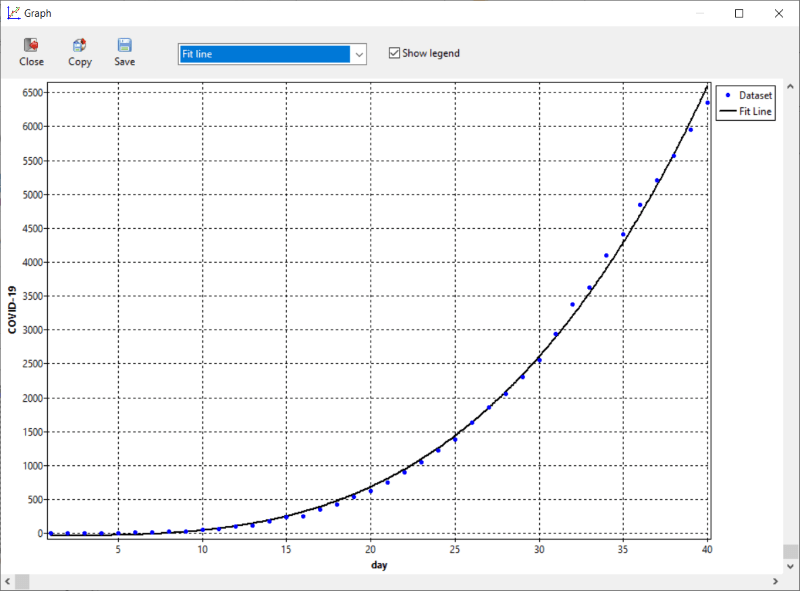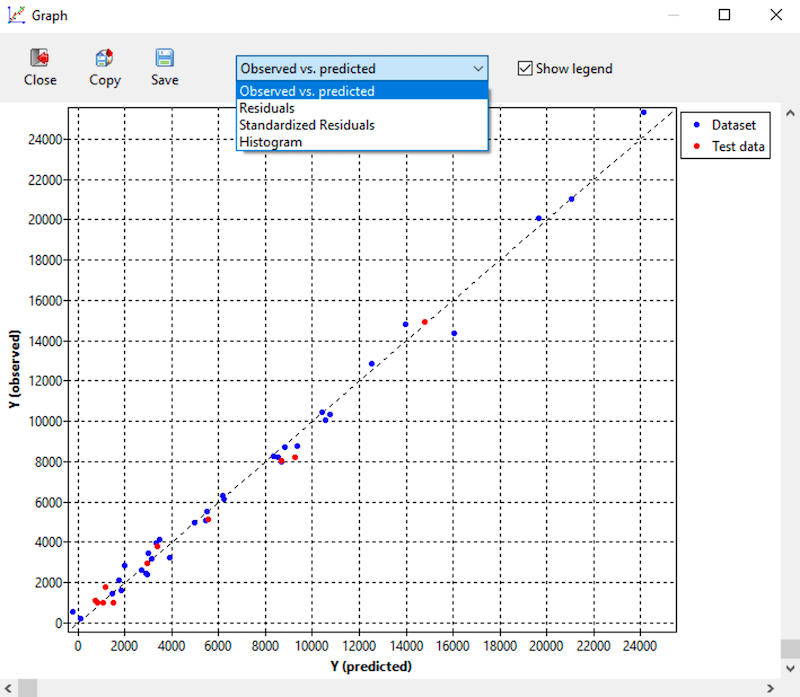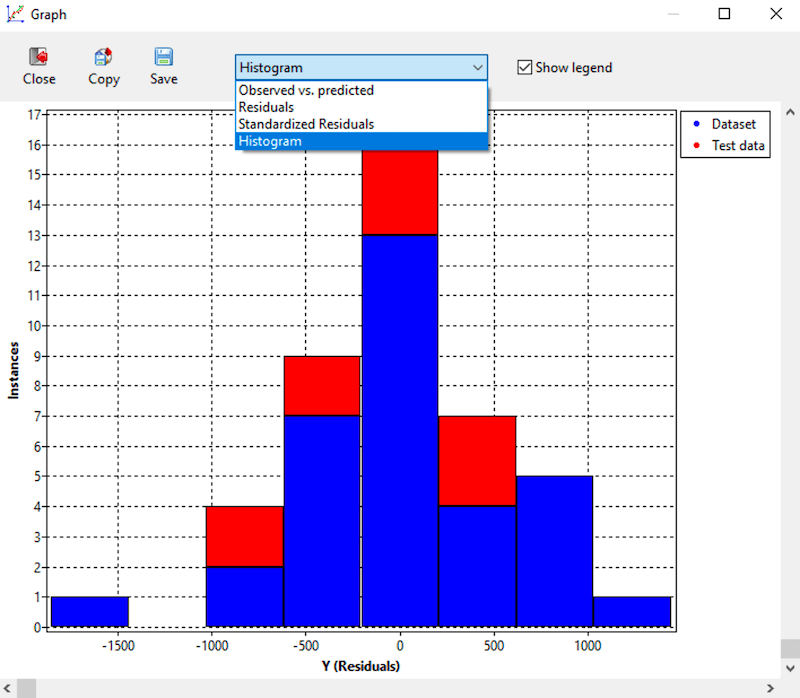# Results

ndCurveMaster lets you automatic fit a set of different models to your data, for example: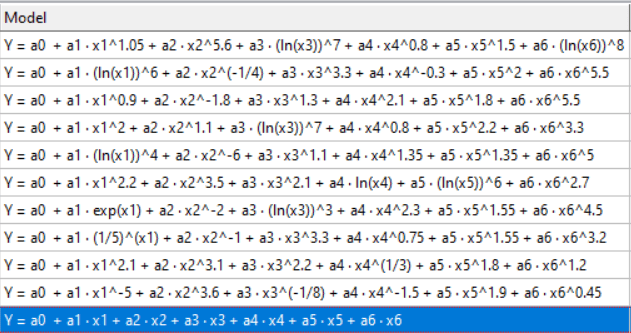This program offers the development of a regression model for any number of input variables without any restrictions.
These models can be developed using full automatic search or manually by the user. The following types of model may be automatically discovered:

1. Multiple Nonlinear Regression (MNLR) model, for example:
`Y = a0 + a1 · exp(x1)^-0.5 + a2 · (ln(x2))^8 + a3 · exp(x3)^1.5 + a4 · x4^4.1 + a5 · x5^5 + a6 · x6^6`
2. MLNR model with variable combination, for example:
`Y = a0 + a1 · exp(x1)^-0.1 + a2 · x2 + a3 · x3 + a4 · x4^(1/8) + a5 · x1^0.9 · x2^0.9 + a6 · x1^1.35 · x3 + a7 · (ln(x1))^5 · ln(x4) + a8 · x2 · x3 + a9 · (ln(x3))^8 · x4^0.55 + a10 · x1^1.25 · x2 · x3 + a11 · x2^(1/2) · x3^6 · x4^0.8`
3. Any order Polynomial Regression Model, for example:
`Y = a0 + a1 · x1 + a2 · x2 + a3 · x3 + a4 · x1 · x2 + a5 · x1 · x3 + a6 · x2 · x3 + a7 · x1 · x2 · x3 + a8 · x1^2 + a9 · x2^2 + a10 · x3^2 + a11 · x1^3 + a12 · x2^3 + a13 · x3^3 + a14 · x1^2 · x2 + a15 · x1 · x2^2 + a16 · x1^2 · x3 + a17 · x1 · x3^2 + a18 · x2 · x3^2 + a19 · x2^2 · x3 + a20 · x1^2 · x2 · x3 + a21 · x1 · x2^2 · x3 + a22 · x1 · x2 · x3^2`
4. Regression model consisting of only input variable combination, for example:
`Y = a1 · x1^0.1 · x2^0.2 · x3^(-1/12) · x4^(-1/12) · x5^5`

ndCurveMaster offers manually develop a regression model by using the knowledge and experience of the user. The following models may be developed by using this method:

1. Non-linear model of any form, for example:
`Y = a0 + a1 · (ln(x1))^8 + a2 · (ln(x2))^7 + a3 · exp(x3)^1.5 + a4 · x4^-11 + a5 · x5^5`
2. logarithmic transformated models, such as:
• Linear-log model, for example:
`Y = a0 + a1 · ln(x1) + a2 · ln(x2) + a3 · ln(x3) + a4 · ln(x4) + a5 · ln(x5)`
• Log-linear model, for example:
`ln(Y) = a0 + a1 · x1 + a2 · x2 + a3 · x3 + a4 · x4 + a5 · x5`
• Log-log model, for example:
`ln(Y) = a0 + a1 · ln(x1) + a2 · ln(x2) + a3 · ln(x3) + a4 · ln(x4) + a5 · ln(x5)`
3. Multiple linear regression model (MLR), for example:
`Y = a0 + a1 · x1 + a2 · x2 + a3 · x3 + a4 · x1 · x2 + a5 · x1 · x3 + a6 · x2 · x3 + a7 · x1 · x2 · x3`

• contain the intercept value: `Y = a0 + a1 · x1 + ...`
• not contain the intercept value: `Y = a1 · x1 + ...`

Each of developed model may be freely expanded or reduced. This expansion or reduction may be carried out automatically or manually by the user. In this way, the user can freely modify any model and fit it to data really accurately.
ndCurveMaster has advanced features which allow to check and improve the reliability of developed models, such as:

• Analysis of variance (ANOVA):• Regression analysis:• Multicollinearity prevent and detection: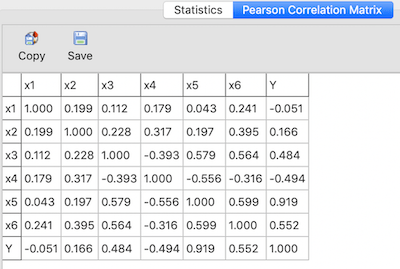Detailed information can be found here

• Overfitting detection:Detailed information can be found here

ndCurveMaster quickly generates high quality graphs as follows: